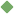factorization 1. [ noun ] (mathematics) the resolution of an integer or polynomial into factors such that when multiplied together they give the integer or polynomial Synonyms: factoring factorisation Related terms: resolution mathematics factor factorize To share this definition press "text" (Facebook, Twitter) or "link" (blog, mail) then paste text link Similar spelling: factorisation   factor_analytic   factor_xiii   factor_viii   factor_xii   factor_vii   factor_out   factor_iii